# 7.3 Graphing linear equations in two variables  (Page 3/3)

 Page 3 / 3

## Practice set a

Graph $3x+y=3$ using the intercept method.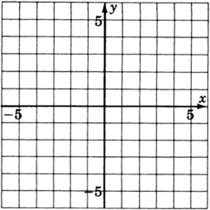When $x=0$ , $y=3$ ; when $y=0$ , $x=1$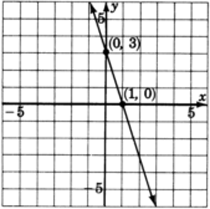## Graphing using any two or more points

The graphs we have constructed so far have been done by finding two particular points, the intercepts. Actually, any two points will do. We chose to use the intercepts because they are usually the easiest to work with. In the next example, we will graph two equations using points other than the intercepts. We’ll use three points, the extra point serving as a check.

## Sample set b

$x-3y=-10$ .
We can find three points by choosing three $x\text{-values}$ and computing to find the corresponding $y\text{-values}$ . We’ll put our results in a table for ease of reading.

Since we are going to choose $x\text{-values}$ and then compute to find the corresponding $y\text{-values}$ , it will be to our advantage to solve the given equation for $y$ .

$\begin{array}{rrrr}\hfill x-3y& \hfill =& -10\hfill & \hfill \text{Subtract}\text{\hspace{0.17em}}x\text{\hspace{0.17em}}\text{from both sides}\text{.}\\ \hfill -3y& \hfill =& \hfill -x-10& \hfill \text{Divide both sides by}-3.\\ \hfill y& \hfill =& \hfill \frac{1}{3}x+\frac{10}{3}& \hfill \end{array}$

 $x$ $y$ $\left(x,\text{\hspace{0.17em}}y\right)$ 1 If $x=1$ , then $y=\frac{1}{3}\left(1\right)+\frac{10}{3}=\frac{1}{3}+\frac{10}{3}=\frac{11}{3}$ $\left(1,\text{\hspace{0.17em}}\frac{11}{3}\right)$ $-3$ If $x=-3$ , then $y=\frac{1}{3}\left(-3\right)+\frac{10}{3}=-1+\frac{10}{3}=\frac{7}{3}$ $\left(-3,\frac{7}{3}\right)$ 3 If $x=3$ , then $y=\frac{1}{3}\left(3\right)+\frac{10}{3}=1+\frac{10}{3}=\frac{13}{3}$ $\left(3,\text{\hspace{0.17em}}\frac{13}{3}\right)$

Thus, we have the three ordered pairs (points), $\left(1,\text{\hspace{0.17em}}\frac{11}{3}\right)$ , $\left(-3,\text{\hspace{0.17em}}\frac{7}{3}\right)$ , $\left(3,\text{\hspace{0.17em}}\frac{13}{3}\right)$ . If we wish, we can change the improper fractions to mixed numbers, $\left(1,3\text{\hspace{0.17em}}\frac{2}{3}\right)$ , $\left(-3,2\text{\hspace{0.17em}}\frac{1}{3}\right)$ , $\left(3,4\text{\hspace{0.17em}}\frac{1}{3}\right)$ .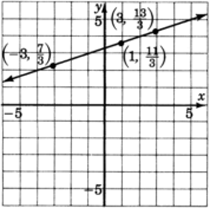$4x+4y=0$

We solve for $y$ .

$\begin{array}{ccc}4y& =& -4x\\ y& =& -x\end{array}$

 $x$ $y$ $\left(x,\text{\hspace{0.17em}}y\right)$ 0 0 $\left(0,\text{\hspace{0.17em}}0\right)$ 2 $-2$ $\left(2,\text{\hspace{0.17em}}-2\right)$ $-3$ 3 $\left(-3,\text{\hspace{0.17em}}3\right)$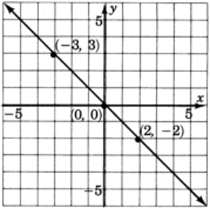Notice that the $x-$ and $y\text{-intercepts}$ are the same point. Thus the intercept method does not provide enough information to construct this graph.

When an equation is given in the general form $ax+by=c$ , usually the most efficient approach to constructing the graph is to use the intercept method, when it works.

## Practice set b

Graph the following equations.

$x-5y=5$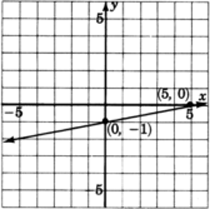$x+2y=6$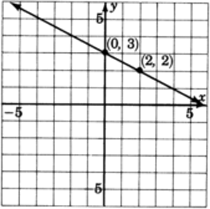$2x+y=1$## Slanted, horizontal, and vertical lines

In all the graphs we have observed so far, the lines have been slanted. This will always be the case when both variables appear in the equation. If only one variable appears in the equation, then the line will be either vertical or horizontal. To see why, let’s consider a specific case:

Using the general form of a line, $ax+by=c$ , we can produce an equation with exactly one variable by choosing $a=0$ , $b=5$ , and $c=15$ . The equation $ax+by=c$ then becomes

$0x+5y=15$

Since $0\cdot \left(\text{any}\text{\hspace{0.17em}}\text{number}\right)=0$ , the term $0x$ is $0$ for any number that is chosen for $x$ .

Thus,

$0x+5y=15$

becomes

$0+5y=15$

But, $0$ is the additive identity and $0+5y=5y$ .

$5y=15$

Then, solving for $y$ we get

$y=3$

This is an equation in which exactly one variable appears.

This means that regardless of which number we choose for $x$ , the corresponding $y\text{-value}$ is 3. Since the $y\text{-value}$ is always the same as we move from left-to-right through the $x\text{-values}$ , the height of the line above the $x\text{-axis}$ is always the same (in this case, 3 units). This type of line must be horizontal.

An argument similar to the one above will show that if the only variable that appears is $x$ , we can expect to get a vertical line.

## Sample set c

Graph $y=4$ .
The only variable appearing is $y$ . Regardless of which $x\text{-value}$ we choose, the $y\text{-value}$ is always 4. All points with a $y\text{-value}$ of 4 satisfy the equation. Thus we get a horizontal line 4 unit above the $x\text{-axis}$ .

 $x$ $y$ $\left(x,\text{\hspace{0.17em}}y\right)$ $-3$ 4 $\left(-3,\text{\hspace{0.17em}}4\right)$ $-2$ 4 $\left(-2,\text{\hspace{0.17em}}4\right)$ $-1$ 4 $\left(-1,\text{\hspace{0.17em}}4\right)$ 0 4 $\left(0,\text{\hspace{0.17em}}4\right)$ 1 4 $\left(1,\text{\hspace{0.17em}}4\right)$ 2 4 $\left(2,\text{\hspace{0.17em}}4\right)$ 3 4 $\left(3,\text{\hspace{0.17em}}4\right)$ 4 4 $\left(4,\text{\hspace{0.17em}}4\right)$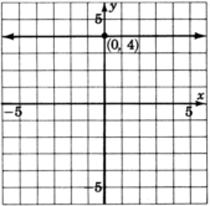Graph $x=-2$ .
The only variable that appears is $x$ . Regardless of which $y\text{-value}$ we choose, the $x\text{-value}$ will always be $-2$ . Thus, we get a vertical line two units to the left of the $y\text{-axis}$ .

 $x$ $y$ $\left(x,\text{}y\right)$ $-2$ $-4$ $\left(-2,\text{\hspace{0.17em}}-4\right)$ $-2$ $-3$ $\left(-2,\text{\hspace{0.17em}}-3\right)$ $-2$ $-2$ $\left(-2,\text{\hspace{0.17em}}-2\right)$ $-2$ $-1$ $\left(-2,\text{\hspace{0.17em}}-1\right)$ $-2$ 0 $\left(-2,\text{\hspace{0.17em}}0\right)$ $-2$ 1 $\left(-2,\text{\hspace{0.17em}}1\right)$ $-2$ 2 $\left(-2,\text{\hspace{0.17em}}0\right)$ $-2$ 3 $\left(-2,\text{\hspace{0.17em}}3\right)$ $-2$ 4 $\left(-2,\text{\hspace{0.17em}}4\right)$
•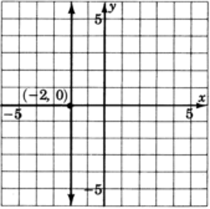## Practice set c

Graph $y=2$ .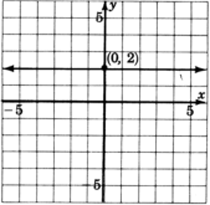Graph $x=-4$ .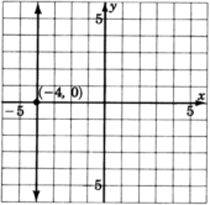## Summarizing our results we can make the following observations:

1. When a linear equation in two variables is written in the form $ax+by=c$ , we say it is written in general form .
2. To graph an equation in general form it is sometimes convenient to use the intercept method.
3. A linear equation in which both variables appear will graph as a slanted line.
4. A linear equation in which only one variable appears will graph as either a vertical or horizontal line.

$x=a$ graphs as a vertical line passing through $a$ on the $x\text{-axis}$ .
$y=b$ graphs as a horizontal line passing through $b$ on the $y\text{-axis}$ .

## Exercises

For the following problems, graph the equations.

$-3x+y=-1$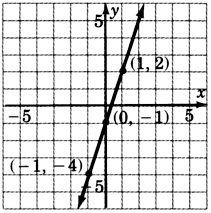$3x-2y=6$$-2x+y=4$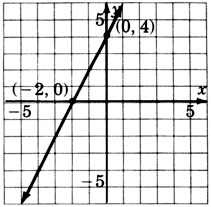$x-3y=5$$2x-3y=6$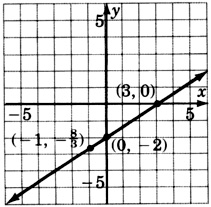$2x+5y=10$$3\left(x-y\right)=9$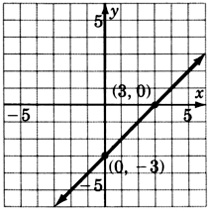$-2x+3y=-12$$y+x=1$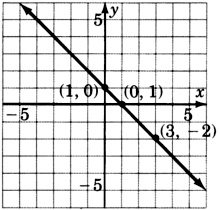$4y-x-12=0$$2x-y+4=0$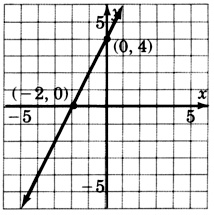$-2x+5y=0$$y-5x+4=0$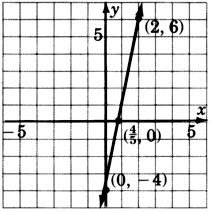$0x+y=3$$0x+2y=2$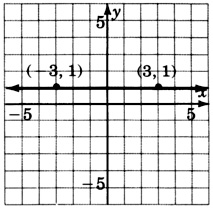$0x+\frac{1}{4}y=1$$4x+0y=16$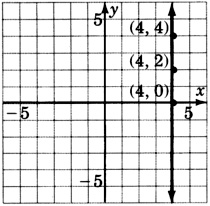$\frac{1}{2}x+0y=-1$$\frac{2}{3}x+0y=1$$x=\frac{3}{2}$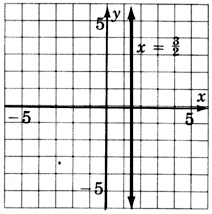$y=3$$y=-2$$y=-2$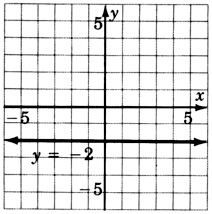$-4y=20$$x=-4$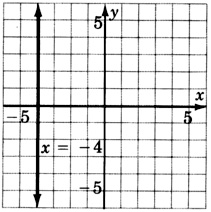$-3x=-9$$-x+4=0$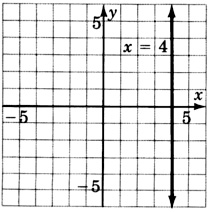Construct the graph of all the points that have coordinates $\left(a,\text{\hspace{0.17em}}a\right)$ , that is, for each point, the $x-$ and $y\text{-values}$ are the same.##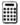Calculator problems

$2.53x+4.77y=8.45$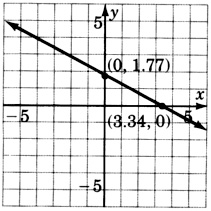$1.96x+2.05y=6.55$$4.1x-6.6y=15.5$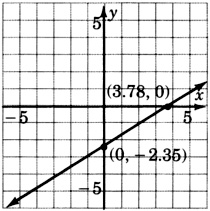$626.01x-506.73y=2443.50$## Exercises for review

( [link] ) Name the property of real numbers that makes $4+x=x+4$ a true statement.

( [link] ) Supply the missing word. The absolute value of a number $a$ , denoted $|a|$ , is the from $a$ to $0$ on the number line.

( [link] ) Find the product $\left(3x+2\right)\left(x-7\right)$ .

$3{x}^{2}-19x-14$

( [link] ) Solve the equation $3\left[3\left(x-2\right)+4x\right]-24=0$ .

( [link] ) Supply the missing word. The coordinate axes divide the plane into four equal regions called .

where we get a research paper on Nano chemistry....?
nanopartical of organic/inorganic / physical chemistry , pdf / thesis / review
Ali
what are the products of Nano chemistry?
There are lots of products of nano chemistry... Like nano coatings.....carbon fiber.. And lots of others..
learn
Even nanotechnology is pretty much all about chemistry... Its the chemistry on quantum or atomic level
learn
da
no nanotechnology is also a part of physics and maths it requires angle formulas and some pressure regarding concepts
Bhagvanji
hey
Giriraj
Preparation and Applications of Nanomaterial for Drug Delivery
revolt
da
Application of nanotechnology in medicine
what is variations in raman spectra for nanomaterials
ya I also want to know the raman spectra
Bhagvanji
I only see partial conversation and what's the question here!
what about nanotechnology for water purification
please someone correct me if I'm wrong but I think one can use nanoparticles, specially silver nanoparticles for water treatment.
Damian
yes that's correct
Professor
I think
Professor
Nasa has use it in the 60's, copper as water purification in the moon travel.
Alexandre
nanocopper obvius
Alexandre
what is the stm
is there industrial application of fullrenes. What is the method to prepare fullrene on large scale.?
Rafiq
industrial application...? mmm I think on the medical side as drug carrier, but you should go deeper on your research, I may be wrong
Damian
How we are making nano material?
what is a peer
What is meant by 'nano scale'?
What is STMs full form?
LITNING
scanning tunneling microscope
Sahil
how nano science is used for hydrophobicity
Santosh
Do u think that Graphene and Fullrene fiber can be used to make Air Plane body structure the lightest and strongest. Rafiq
Rafiq
what is differents between GO and RGO?
Mahi
what is simplest way to understand the applications of nano robots used to detect the cancer affected cell of human body.? How this robot is carried to required site of body cell.? what will be the carrier material and how can be detected that correct delivery of drug is done Rafiq
Rafiq
if virus is killing to make ARTIFICIAL DNA OF GRAPHENE FOR KILLED THE VIRUS .THIS IS OUR ASSUMPTION
Anam
analytical skills graphene is prepared to kill any type viruses .
Anam
Any one who tell me about Preparation and application of Nanomaterial for drug Delivery
Hafiz
what is Nano technology ?
write examples of Nano molecule?
Bob
The nanotechnology is as new science, to scale nanometric
brayan
nanotechnology is the study, desing, synthesis, manipulation and application of materials and functional systems through control of matter at nanoscale
Damian
Is there any normative that regulates the use of silver nanoparticles?
what king of growth are you checking .?
Renato
What fields keep nano created devices from performing or assimulating ? Magnetic fields ? Are do they assimilate ?
why we need to study biomolecules, molecular biology in nanotechnology?
?
Kyle
yes I'm doing my masters in nanotechnology, we are being studying all these domains as well..
why?
what school?
Kyle
biomolecules are e building blocks of every organics and inorganic materials.
Joe
Please keep in mind that it's not allowed to promote any social groups (whatsapp, facebook, etc...), exchange phone numbers, email addresses or ask for personal information on QuizOver's platform.

#### Get Jobilize Job Search Mobile App in your pocket Now!ByByByBy Inderjeet BrarBy Cath YuBy Rohini AjayBy OpenStaxBy John GabrieliBy OpenStaxBy Brooke DelaneyBy Kevin MoquinBy Yacoub Jayoghli